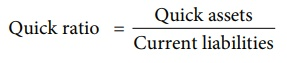Home | | Accountancy 12th Std | Liquidity ratios

# Liquidity ratios

Liquidity means capability of being converted into cash with ease. Liquidity ratios help to assess the ability of a business concern to meet its short term financial obligations.

Liquidity ratios

Liquidity means capability of being converted into cash with ease. Liquidity ratios help to assess the ability of a business concern to meet its short term financial obligations. Short term assets (current assets) are more liquid as compared to long term assets (fixed assets). Liquidity ratios are also called as short term solvency ratios.

Liquidity ratios include: (i) Current ratio and (ii) Quick ratio.

## (i) Current ratio

Current ratio gives the proportion of current assets to current liabilities of a business concern. It is computed by dividing current assets by current liabilities. Current ratio indicates the ability of an entity to meet its current liabilities as and when they are due for payment. It is calculated as follows:Higher the current ratio, the better is the liquidity position, as the firm will be in a better position to pay its current liabilities. However, a much higher ratio may indicate inefficient investment policies of the management.

Illustration 1

Calculate current ratio from the following information:SolutionCurrent assets = Current investments + Inventories + Trade receivables + Cash and cash equivalents + Prepaid expenses

= 80,000 + 1,60,000 + 4,00,000 + 1,20,000 + 40,000 = ₹ 8,00,000

Current liabilities = Trade creditors +Bills payable + Expenses payable

= 1,60,000 + 1,00,000 + 1,40,000 = ₹ 4,00,000

## (ii) Quick ratio

Quick ratio gives the proportion of quick assets to current liabilities. It indicates whether the business concern is in a position to pay its current liabilities as and when they become due, out of its quick assets. Quick assets are current assets excluding inventories and prepaid expenses. It is otherwise called liquid ratio or acid test ratio. It is calculated as follows:

Quick ratio = Quick assets / Current liabilitiesQuick assets = Current assets – Inventories – Prepaid expenses

Higher the quick ratio, better is the short-term financial position of an enterprise.

Illustration 2

Calculate quick ratio of Ananth Constructions Ltd from the information given below.SolutionQuick assets = Current assets – Inventories – Prepaid expenses

= 2,50,000 – 50,000 – 15,000

= ₹ 1,85,000

Illustration 3

Following is the balance sheet of Magesh Ltd. as on 31st March, 2019:Calculate: (i) Current ratio (ii) Quick ratio

Solution

(i) Current ratio = Current assets/Current liabilities = 1,50,000/50,000 = 3:1

Current assets = Inventories + Trade receivables + Cash and cash equivalents + Prepaid expenses

= 45,000 + 70,000 + 30,000 + 5,000 = ₹ 1,50,000

Current liabilities = Short term borrowings + Trade payables + Expenses payable  + Short term provisions

= 17,000 + 25,000 + 3,000 + 5,000 = ₹ 50,000

(ii) Quick ratio = Quick assets / Current liabilities

= 1,00,000/50,000 = 2:1

Quick assets = Total current assets – Inventories – Prepaid expenses

= 1,50,000 – 45,000 – 5,000 = ₹ 1,00,000

Tags : Computation of ratios | Accountancy , 12th Accountancy : Chapter 9 : Ratio Analysis
Study Material, Lecturing Notes, Assignment, Reference, Wiki description explanation, brief detail
12th Accountancy : Chapter 9 : Ratio Analysis : Liquidity ratios | Computation of ratios | Accountancy